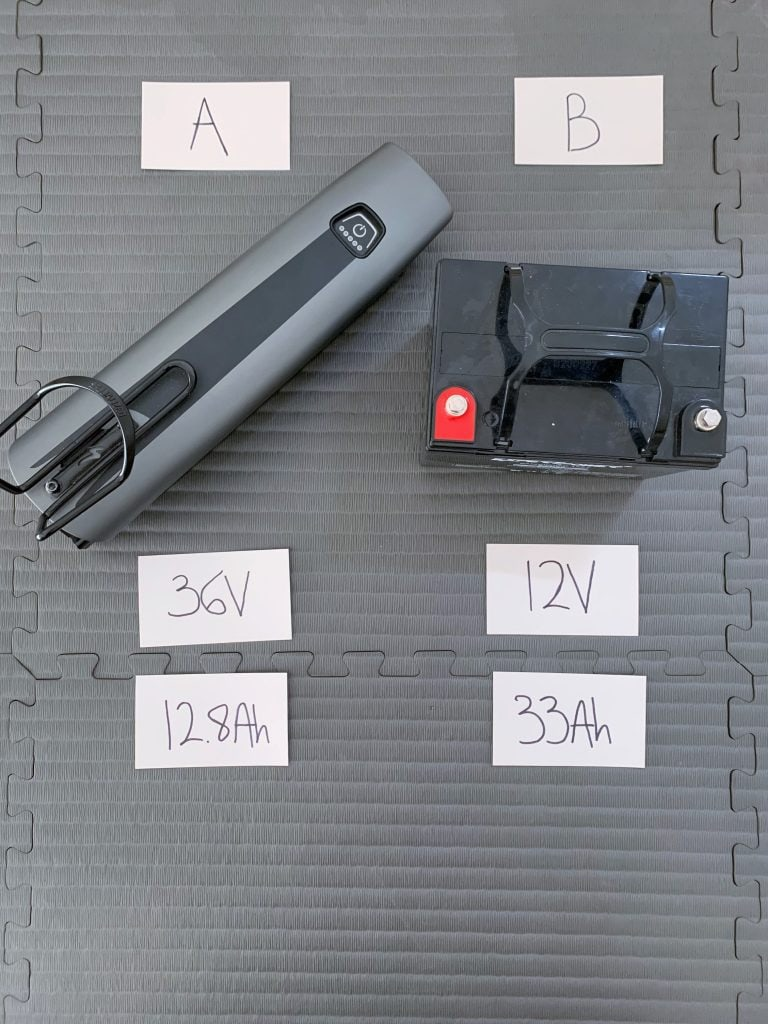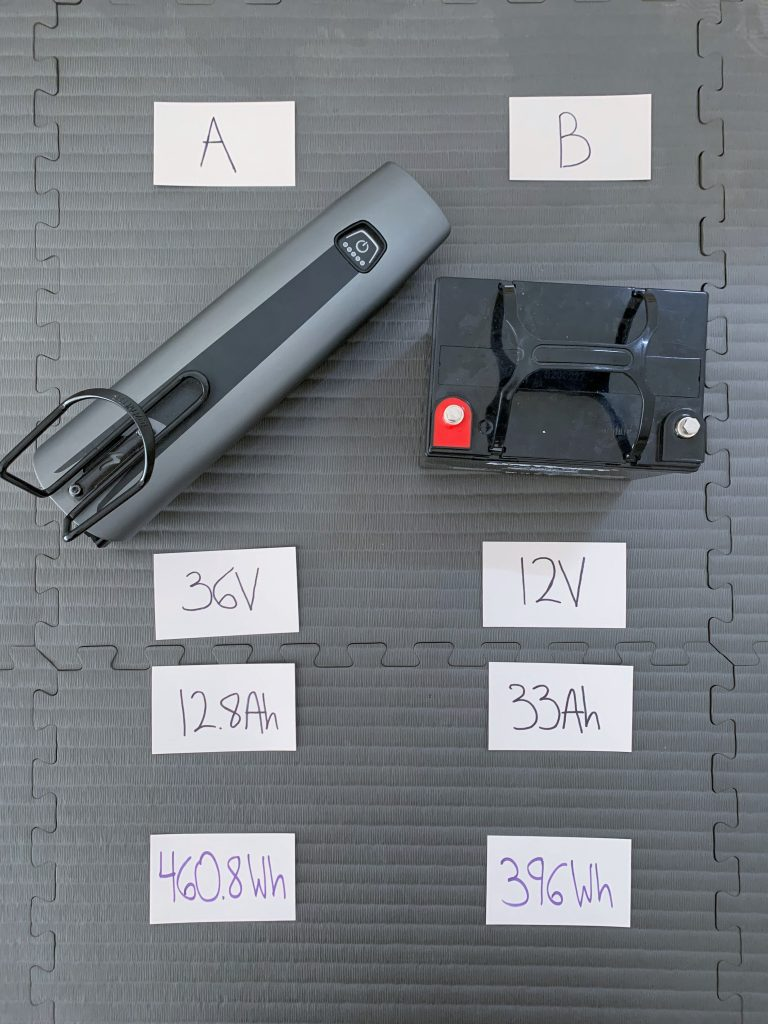# Amp Hours to Watt Hours (Ah to Wh) Conversion Calculator

## Amp Hours to Watt Hours Conversion Calculator

Error: Enter a valid number of amp hours (Ah).
Error: Enter a valid voltage (V).
watt hours (Wh)
Conversion formula: Wh = Ah × V

## How to Convert Amp Hours to Watt Hours (Ah to Wh)

To convert amp hours to watt hours, simply multiply amp hours by volts:

`Watt hours (Wh) = Amp hours (Ah) × Volts (V)`

### Example

Let's say you have a 12V battery with a 50Ah capacity.

To calculate your battery's watt hours, you'd multiply 50Ah × 12V:

`50 amp hours (Ah) × 12 volts (V) = 600 watt hours (Wh)`

In this example, your battery has a capacity of 600Wh.

Easy!

### Why Convert Amp Hours to Watt Hours?

Converting amp hours to watt hours makes it easier to compare how much energy different batteries can deliver.

The total energy a battery can provide depends on both its amp hours and voltage, so only comparing amp hours can lead you to some erroneous conclusions.

For example, consider these two batteries of mine, which I'll call Battery A and Battery B:Battery A (left) is a 36V 12.8Ah battery, while Battery B is a 12V 33Ah battery. If you were to only compare their amp hours, you'd assume Battery B has a greater capacity.

Battery A is a 36V 12.8Ah battery, while Battery B is a 12V 33Ah battery.

If you only looked at amp hours, you'd assume Battery B can provide a lot more energy.

After all, 33Ah is much greater than 12.8Ah.

But these batteries have different voltages, so only comparing their amp hours is like comparing apples to oranges. We need to take voltage into account for both batteries, and we do that by calculating their watt hours.

Once we do, we can make an apples to apples comparison of the amount of energy they can provide. And when we do, we see how wrong we were:However, when we calculate each battery's capacity in watt hours, we find that Battery A actually has a greater capacity than Battery B.

That's right, Battery A actually has a greater capacity in watt hours than Battery B.

Why is this the case?

Electricity can be thought of like water flowing through a pipe. Voltage is like the water pressure, while amperage is like the flow rate.

Although Battery A can output its electrons at a higher 'flow rate', they flow at a lower 'pressure'.

On the other hand, Battery B can output its electrons at a lower flow rate, but they flow at three times the pressure. The extra pressure more than makes up for the smaller flow rate, meaning that Battery B ends up delivering more energy.

## How to Convert Watt Hours to Amp Hours (Wh to Ah)

To convert watt hours to amp hours, simply divided watt hours by volts.

`Amp hours (Ah) = Watt hours (Wh) ÷ Volts (V)`

### Example

There are some types of batteries, such as solar generators, that by convention tend to describe their capacity in watt hours.

For instance, let's say you own the Jackery Explorer 1500, a solar generator with a 1534Wh capacity.

To calculate your generator's amp hours, you first need to find its battery voltage. You look online and see that the Explorer 1500 has a 25.2V battery.

With these two pieces of info, you can find its amp hours using the above equation:

`1534Wh ÷ 25.2V = 60.9Ah`

In this example, your solar generator has a capacity of 60.9Ah.

## How to Convert Amp Hours to Kilowatt Hours (Ah to kWh)

1 kilowatt hour is equal to 1000 watt hours. (Just like how 1 kilometer is 1000 meters.)

So, to convert amp hours to kilowatt hours, you first convert the amp hours to watt hours and then divide by 1000.

`Kilowatt hours (kWh) = Amp hours (Ah) × Volts (V) ÷ 1000`

### Example

Let's say you own a 12V 100Ah lithium battery. To convert its amp hours to kilowatt hours, you'd do the following:

`12V × 100Ah ÷ 1000 = 1.2kWh`

Your lithium battery has a 1.2kWh capacity. That's some serious power!

## How to Convert Milliamp Hours to Watt Hours (mAh to Wh)

1 amp hour is equal to 1000 milliamp hours. (Just like how 1 meter is 1000 millimeters.)

So, to convert milliamp hours to watt hours, you multiply millamp hours by volts and then divide by 1000.

`Watt hours (Wh) = Milliamp hours (mAh) × Volts (V) ÷ 1000`

### Example

Let's say your phone has a 3.7V 3000mAh battery.

To calculate its capacity in watt hours, you'd do the following:

`3000mAh × 3.7V ÷ 1000 = 11.1Wh`

In this example, your phone battery has a 11.1Wh capacity.

## More Solar Calculators